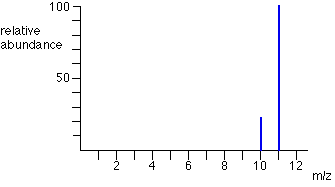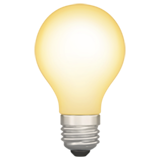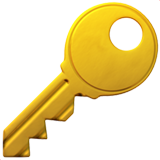Unit 2

# The Atomic Theory of Matter

Between 1803 and 1807, John Dalton created an atomic theory.

For example, his theory can be used to deduce the law of multiple proportions:

If two elements A and B combine to form more than one compound, the masses of B that can combine with a given mass of A are in the ratio of small whole numbers.

Compounds are formed when atoms of more than one element combine. A given compound always has the same relative number and kind of atoms.

# The Discovery of Atomic Structure

Particles with the same charge repel one another, while particles with different charges attract one another.

Around 1900, Ernest Rutherford found that there are three types of radiation:

• alpha
• path gets bent by electric field
• fast-moving particles
• positive charge of 2+ (attracted to negative plate)
• beta
• path gets bent by electric field
• fast-moving particles
• high-speed electrons
• negative charge of 1- (attracted to positive plate)
• gamma
• path does not get bent by electric field
• high energy radiation (similar to X-rays)
• does not consist of particles with mass
• carries no charge

Rutherford discovered that, when sending a beam of alpha particles through a piece of gold foil, only very few scatter, and a few at very large angles. This added evidence for the nuclear model of the atom, where positive charge resides in the small and dense region (the nucleus) and the rest of the volume of an atom is empty space in which electrons move around the nucleus. Most of the time, the alpha particles were simply passing through empty space.

Later experiments led to the discovery of positive particles (protons) and negative neutral particles (neutrons).

# The Modern View of Atomic Structure

The charge of an proton or electron is (positive or negative) 1.602 * 10^-19 C (coulomb, the SI unit for electrical charge). However, for convenience, this value is given the electrical charge of "1".

Most of the mass of an atom comes from the nucleus, because it contains the protons and neutrons. The mass unit used is atomic mass unit (amu), where 1 amu = 1.66054 * 10 ^ -24g.

The number of protons in an atom of any element is called that element's atomic number. Atoms have no net electrical charge, so this is also the number of electrons it contains. However, the number of neutrons may vary (called isotopes of one another), as in this example of carbon:

$^{14}_{6}\,\text{C}$

This is "carbon fourteen", and represents that this atom has a mass of 14 and atomic number of 6. Therefore, it has 14 - 6 = 8 neutrons. The 6 may be omitted, since it's the standard atom number (number of protons) for carbon.

# Atomic Weights

Today, the atomic mass unit is defined by assigning a mass of 12 amu to a chemically unbound atom of carbon-12.

Most elements occur in nature as various isotopes. The average atomic mass of an element, or the element's atomic weight, is determined by summing the masses of isotopes multiplied by their relative abundances (a weighted average).

The mass spectrometer is the most accurate way for determining atomic weights. A gaseous sample is introduced into the system, and bombarded by a stream of high-energy electrons. Collisions between these electrons and the sample produce positively charged ions, which are then accelerated towards a negatively charged grid. After the ions pass through the grid, they encounter two slits that allow only a narrow beam of ions to pass. As the beam passes through the poles of the magnet, they are deflected on a curved path. The more mass an ion has, the less it is deflected.

The strength of the magnetic field or the voltage of the grid can be changed, so the ions are propelled into a detector at the other end of the system.

## Mass spectrum

A mass spectrum is a graph showing the relative abundance of different isotopes of an element (how often elements with different masses appear in nature).

This is an example of a mass spectrum:From the peaks on this mass spectrum, one can conclude that the average mass of this element is slightly less than 11 amu, since most samples were measured to be an isotope with mass 11. However, some samples were slighly lighter (10 amu), which drags the average slightly down.

# The Periodic Table

When ordering elements in order of increasing atomic number (number of protons), their chemical and physical properties show a repeating, or periodic, pattern.

## Periodic table terminology

• period — horizontal row groups

• groups — vertical column groups

• Elements on the left of the periodic table, other than hydrogen, are metals.

• Share characteristic properties such as luster and high electrical and heat conductivity.
• All except mercury (Hg) are solid at room temperature.
• Elements on the right side are nonmetals.

• At room temperature some are gaseous, some are solid, and one is liquid.
• Separating the two groups in the table are metalloids, which have properties that fall between metals and nonmetals.

# Molecules and Molecular Compounds

Only noble-gas elements are normally found in nature as single atoms. Most matter is composed of molecules or ions.

Hydrogen, oxygen, nitrogen, and the halogens (H2, O2, N2, F2, Cl2, Br2, and I2) normally occur as diatomic molecules (made up of two atoms).

Molecular compounds — compounds composed of more than one type of atom in several molecules.

## Formulas and models

• Molecular formula — indicates the actual number of atoms in a molecule (e.g. C6H12O6)
• Empirical formula — gives only the relative number of atoms of each type in a molecule (e.g. CH2O)
• These are used because certain common methods of analyzing substances lead to the empirical formula only
• Additional experiments can give the information needed to convert the empirical formula to the molecular one
• Structural formula — shows which atoms are attached to which using letters and lines in between
• Ball-and-stick models — show atoms as spheres and bonds as sticks
• Has advantage of being able to accurately represent the angles at which atoms are attached to one another
• Space-filling model — shows atoms and spheres directly attached to each other
• Depicts what a molecule would look like if the atoms were scaled up in size

# Ions and Ionic Compounds

## Definitions

• ion — an atom when electrons are added or removed
• cation — positively charged ion
• anion — negatively charged ion
• ionic compound — a compound made up of cations and anions

Net charges of an ion are represented by a superscript (e.g. 2+ or 3-)

The noble-gas elements (group 8A) are chemically nonreactive elements that form very few compounds. Therefore, many elements gain or lose electrons to end up with the same number of electrons as the noble gasses closest to them, because these numbers are the most stable.

Generally, cations are metal ions and anions are nonmetal ions (due to their placement on the periodic table in relation to noble gasses).Ionic compounds are generally compounds of metals and nonmetals, as in NaCl. Molecular compounds are generally composed of nonmetals only, such as H20.

Ions in ionic compounds are arranged in 3D structures. There is no discrete "molecule" of NaCl, so we can only write an empirical formula for it. This holds true for most other ionic compounds.

Chemical compounds are always electrically neutral, so the ions in an ionic compound always occur in such a ratio so that the total positive and negative charges are equal.When balancing an ionic compound, if the charges on the cation and anion are equal, the subscript on each ion is 1. If the charges are not equal, the subscripts and superscripts flip. Example below.
$3\text{Mg}^{2+} + 2\text{N}^{3-} → \text{Mg}_{3}\text{N}_{2}$

# Naming Inorganic Compounds

## Cations

Cations formed from metal atoms have the same name as the metal.

• Na+ = sodium ion
• (Zn)2+ = zinc ion

If a metal can form cations with different charges, the positive charge is indicated by a Roman numeral in parenthesis after the name of the metal.

• (Fe)2+ = iron(II) ion
• (Fe)3+ = iron(III) ion

An older method for indicating the amount of positive charge uses -ous and -ic endings added to the root of the element's Latin name.

• (Fe)2+ = ferrous ion
• (Fe)3+ = ferric ion
• Cu+ = caprous ion
• (Cu)2+ = cupric ion

Cations formed from nonmetal atoms have names that end in -ium.

• (NH4)+ = ammonium ion
• (H3)0+ = hydronium ion

## Anions

Monatomic anions are named by replacing the ending of the name of the element with -ide.

• H- = hydride ion
• (O)2- = oxide ion

A few polyatomic anions also have names ending in -ide.

• OH- = hydroxide ion
• CN- = cyanide ion

Polyatomic anions containing oxygen have names ending in either -ate or -ite and are called oxyanions. The -ate is used for the most common or representative oxyanion of an element, and -ite is used for an oxyanion that has the same charge but one oxygen atom fewer.

• (NO3)- = nitrate ion
• (NO2)- = nitrite ion

Prefixes are used when the series of oxyanions of an element extends to four members (like with the halogens). The prefix per- indicates one or more O atom than -ate; hypo- indicates one O atom fewer than -ite.

• (ClO4)- = perchlorate ion
• (ClO3)- = chlorate ion
• (ClO2)- = chlorite ion
• (ClO)- = hypochlorite ion

Anions derived by adding H+ to an oxyanion are named by adding as a prefix the word hydrogen or dihydrogen.

• (CO3)2- = carbonate ion
• (HCO3)- = hydrogen carbonate ion

## Ionic compounds

Names of ionic compounds consist of the cation name followed by the anion name (metal then nonmetal).

## Acids

Acids are an important class of hydrogen-containing compounds, whose molecules yield hydrogen ions (H+) when dissolved in water. An acid is composed of an anion connected to enough H+ ions to neutralize the anion's charge.

The name of an acid is related to the name of its anion.

Acids containing anions whose names end in -ide are named by changing the -ide ending to -ic, adding the prefix hydro- to the anion name, and then following with the word acid.

• Cl- (chloride) ⇒ HCl (hydrocloric acid)
• (S)2- (sulfide) ⇒ H2S (hydrosulfuric acid)

Acids containing anions whose names end in -ate or -ite are named by changing -ate to -ic and -ite to -ous and then adding the word acid. Prefixes in the anion name are retained in the name of the acid.

• (ClO4)- (perchlorate) ⇒ HclO4 (perchloric acid)
• (ClO2)- (chlorite) ⇒ HclO2 (chlorous acid)You can memorize it using the phrase, "Natick has acid disease."

ate ⇒ ic (Natick)

ite ⇒ ous (like -itis, used to name diseases)

## Binary Molecular Compounds

A binary molecular compound has two elements, and is named similarly to ionic compounds.

1. The name of the element farther to the left in the periodic table (closer to metals) is written first.
• Except when the compound contains oxygen and chlorine, bromine, or iodine, in wich case oxygen is written last.
2. If both elements are in the same group, the one closer to the bottom of the table is named first.
3. The name of the second element is given an -ide ending.
4. Greek prefixes indicate the number of atoms of each element
• Except mono- is never used.

# Some Simple Organic Compounds

The study of compounds of carbon is called organic chemistry.

Compounds that contain only carbon and hydrogen are called hydrocarbons. In the simplest class of hydrocarbons, alkanes, each carbon is bonded to four other atoms. The three smallest alkanes are methane (CH4), ethane (C2H6), propane (C3H8), and butane (C4H10). Each alkane has a name that ends in -ane. For alkanes with five or more carbons, names are derived using standard numeral prefixes.

Other classes of organic compounds are obtained when one or more hydrogen atoms in an alkane are replaced with functional groups. For example, an alcohol is obtained by replacing an H atom of an alkane with an -OH group. The names of alcohols are derived from that of the alkane by adding an -ol ending (methanol, ethanol, 1-propanol).

The prefix "1" in 1-propanol refers to the replacement of an OH instead of an H occurred at one of the "outer" carbon atoms. Replacement at a "middle" carbon atom would be called 2-propanol or isopropyl alcohol.

1-propanol and 2-propanol have the same molecular formula but different arrangements of atoms. Therefore, they are called isomers, specifically structural isomers.

Much of the richness of organic chemistry comes from the fact that organic compounds can form long chains of carbon-carbon bonds. If tens of thousands of carbon atoms are used to form an alkane, polyethylene is created, which is used to create plastic products.